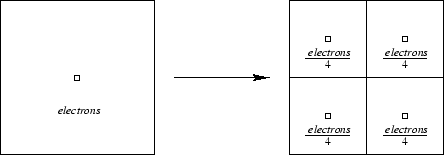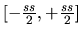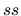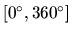Next: 6.4 Stacking of GAIA Up: 6. Simulation and Stacking Previous: 6.2.3 Noise   Contents

# 6.3 Simulation of GAIA BBP Observations

Under our assumptions, the simulation of a GAIA BBP observation on the basis of an HST WFPC2 image essentially involves translation and rotation of the original image, scaling to GAIA exposure time and rebinning into GAIA samples of HST WFPC2 electron counts, image smearing due to GAIA PSF and noise. Step by step, the procedure for the generation of a single simulated observation consists of the following steps:
1. Retrieval of HST electron counts: HST data number counts are retrieved from the fits file provided by the HDA and then converted to HST electron counts using the analog-to-digital conversion gain taken from [Biretta et al. 1996].
2. Subpixeling of HST image: in order to partly recover the resolution of the HST image which would otherwise be lost due to the undersampling of the PSF, each HST pixel is considered as consisting of a mosaic of four square subpixels, containing'' one fourth of the pixel's electron counts each and whose centers are displaced from the pixel's center as shown in Figure 6.4.3. Conversion to GAIA electron counts: GAIA electron counts for a single scan are calculated from HST electron counts by taking into account the different exposure time, and assuming the same electron count rate for the two instruments (see Subsection 6.2.1).
4. Translation and rotation of HST subpixels: since GAIA observation will in general be obtained at a different position and position angle with respect to the original HST image, once the desired observation center and scan direction have been determined the HST subpixels are translated and rotated accordingly.
5. Rebinning of HST subpixels into GAIA samples: each HST subpixel electron count is assigned to the sample containing its center.
6. Convolution with GAIA PSF: the observation is convolved with the PSF described in Subsection 6.2.2. The convolution is computed using a Fourier transform technique, namely calculating the product of the Fourier transforms of the observation and the PSF and then calculating the inverse Fourier transform of the result. Note that the PSFs obtained in Subsection 6.2.2 are sampled with a step of 1/4 pixel along both directions, and therefore need to be resampled and renormalized before convolution.
7. Noise addition: to simulate signal noise we calculated a Poisson deviate of the observation, whereas to simulate readnoise we added to it a Gaussian distribution with zero mean and standard deviation equal to GAIA rms readnoise as calculated in Section 5.5.

Following this procedure, a single GAIA BBP observation is simulated. When generating a realistic all-mission set of simulated observations of a given sky region, however, one has to take into account that in general the different observations will have different centers as well as different scan directions. A set of observation centers, with coordinates in general in the range, whereis the adopted sample size along one of the two axes, and the corresponding set of scan directions, with position angles in general in the range, must therefore be generated. The procedure described above can then be applied to each desired combination of center and scan direction. In the simulations presented in Chapter 7 and in Appendix E, a conservative number of 50 scans was assumed. Note that this is the minimum number of times an Astro will scan any sky region during a 5-year mission, according to Figure 2.3. To a first approximation, the observation centers and scan directions can be taken as randomly distributed, but note that while the first assumption is likely to be verified fairly strictly, this may not always be the case for the second one. The consequences of possible preferred scan directions are therefore described in Section 7.5.Next: 6.4 Stacking of GAIA Up: 6. Simulation and Stacking Previous: 6.2.3 Noise   Contents
Mattia Vaccari 2000-12-05